International Journal of Fluid Mechanics & Thermal Sciences
Volume 1, Issue 2, June 2015, Pages: 36-41

Numerical Investigation of Nucleate Boiling Flow in Water Based Bubble Bumps

R. Garma1, M. Bourouis2, A. Bellagi1

1Unit of Thermic and Thermodynamics of the Industrial Processes, National Engineering School of Monastir, University of Monastir, Monastir, Tunisia

2Department of Mechanical Engineering, Universitat Rovira i Virgili, Tarragona, Spain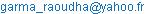(R. Garma)

R. Garma, M. Bourouis, A. Bellagi. Numerical Investigation of Nucleate Boiling Flow in Water Based Bubble Bumps. International Journal of Fluid Mechanics & Thermal Sciences. Vol. 1, No. 2, 2015, pp. 36-41. doi: 10.11648/j.ijfmts.20150102.14

Keywords: Boiling Flow, Bubble Pump, CFD

1. Introduction

In the diffusion absorption refrigerators (DARs) invented by the Swedish engineers von Platen and Munters  in the 1920s, the bubble pump is considered as one of the most important components. Therefore, significant attention has been devoted to this device to improve its performance, which will contribute to the performance of the whole system. The thermally driven bubble pump is a simple vertical tube which can be powered by waste heat or solar thermal energy. When the liquid solution is heated up, vapor bubbles are generated causing pressure difference between the bottom and top of the tube. As result, natural circulation of the liquid solution is driven up through the tube and so boiling flow takes place inside the bubble pump.

Several researches have foxed on numerical investigation of nucleate boiling flow for various applications (thermosyphon, nuclear engineering, PWRs, BWRs, electronics cooling…). CFD modeling of flow and heat transfer in a thermosyphons was carried out by Alizadehdakhel et al. . In this research, the effects of the fill ratio (amount of working fluid) and the heat pipe input were considered. This study shows that the CFD code is a useful tool to model and explain the flow coupled to heat transfer in a thermosyphon. Using the same software CFD code Fluent, numerical simulation of nucleate boiling for power electronics cooling applications was conducted by Narumanchi et al.  to enhance heat removal from electronics packages. Li et al.  modified the basically by incorporating new closure correlations in order to investigate numerically the boiling flow of nitrogen in a vertical tube. In the same way, multidimensional modeling of vertical upward sub-cooled boiling flow was undertaken by Koncar et al. .

In this work a general-purpose CFD code Ansys-Fluent was used for simulations of upward nucleate boiling flow. For modeling the boiling phenomena user defined subroutines (UDFs) are employed. Radial temperature profiles and void fraction distributions for different heat fluxes applied to the stainless-steel made tube wall are discussed.

2. Model Description

Mathematical model utilized in this paper was developed and then applied in CFD codes to be finally implemented in Ansys-Fluent via user-defined functions (UDFs) in conjunction with the Eulerian multiphase model in which the conservation equations are written for each phase, liquid and vapor. The following is a summary of the main model equations for a certain phase q.

2.1. Conservation Equations

The mass conservation equation for q phase is given by: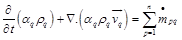(1)

where α is the phase volume fraction of phase q, ρ, the density, v the velocity vector, mpq characterizes the mass transfer rate from phase q to phase p and n, the number of phases.

The momentum conservation equation for q phase is given by: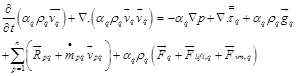(2)

where τ s is the stress-strain tensor, Fpq an interaction force between phases, Fq is an external body force, Flift,q is a lift-force, Fvm,q is a virtual mass force, p is the pressure shared by all phases, and g is the gravitational acceleration vector.

The energy conservation equation for q phase is given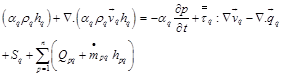(3)

Where h is the specific enthalpy,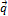is the heat flux vector,is the source term, Q is the intensity of heat exchange between the different phases, and hpq is the difference in the formation enthalpies of phases p and q.

The heat exchange between phases must comply with the local balance conditions, as well as the interfacial mass, momentum interfacial exchange: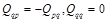(4)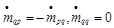(5)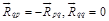(6)

2.2. Mass Equation

The rate of vapor formation per unit volume in Eq. 1 can be written as: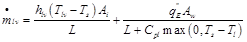(7)

The first term is the mass exchange at the bubble surface and the second term represents the bubble formation due to heat flux at the wall. Here: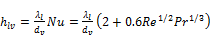(8)

is the interfacial heat transfer coefficient calculated using the Ranz-Marshall correlation, where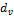is the diameter of the secondary phase (vapor bubble),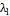is the liquid heat conductivity. Re, Pr and Nu are Reynolds number, Prandtl number and Nusselt number respectively.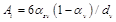(9)

is the interfacial area density, with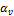is the vapor volume fraction and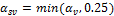;

·= evaporating heat flux calculated from the RPI model [6, 7];

·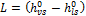= latent heat per unit mass

·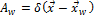= interfacial area density of the wall surface.

Subscripts l, v and s mean liquid phase, vapor phase and saturation state, respectively.

2.3. Momentum Equation

The interfacial drag force between liquid and vapor phases per unit volume is calculated as: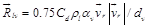(10)

where Cd is the drag coefficient determined by choosing the minimum of the viscous regime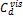and the distorted regime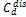: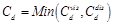(11)

The lift coefficient is calculated as (Moraga et al. ):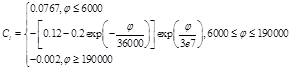(12)

where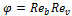,

This lift coefficient combines the opposing action of two lift forces:

Classical aerodynamic lift force resulting from the interaction between bubble and liquid shear,

Lateral force resulting from the interaction between bubble and vortices shed by the bubble wake.

Here,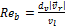is the bubble Reynolds number and,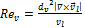is the bubble shear Reynolds number.

2.4. Turbulence Model

The mixture turbulence model, default multiphase turbulence model, was used. It represents the first extension of the single-phase k-ε model. In the present case, using mixture properties and mixture velocities is sufficient to capture important features of the turbulent flow. The equations describing this model are respectively: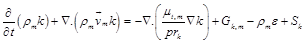(13)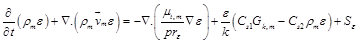(14)

where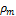and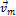are the mixture density and velocity,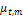is the turbulent viscosity,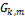is the production rate of turbulence kinetic energy,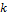is the turbulent kinetic energy,is the dissipation rate.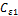and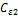are constants.

This model contains two additional terms describing additional bubble stirring and dissipation.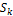is the bubble-induced turbulence in the turbulent kinetic energy equation and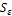the bubble-induced dissipation in the dissipation rate equation: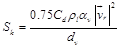(15)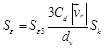(16)

with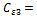0.45

The turbulent diffusion force is calculated as :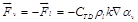(17)

with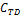is the turbulent dispersion coefficient. This force simulates liquid turbulence induced diffusion of bubbles from the wall into the liquid bulk.

2.5. Wall Boiling Model

According to the basic Rensselaer Polytechnic Institute (RPI) model , the total heat flux from the wall to nucleate boiling consists of three different components, namely the convective heat flux, the quenching heat flux, and the evaporative heat flux: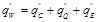(18)

The heated wall surface is subdivided into a portion Ω (0≤ Ω≤1) covered by nucleating bubbles and the rest of the surface area (1-Ω), is covered by liquid.

The convective heat flux is expressed as: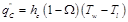(19)

where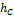is the single phase heat transfer coefficient, and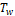and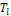are the wall and liquid temperatures, respectively.

The quenching heat flux models the cyclic averaged transient energy transfer related to liquid filling the wall vicinity after bubble detachment, and is expressed as: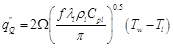(20)

where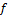is the bubble departure frequency,, the thermal conductivity,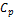, the speciﬁc heat, and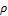, the density.

The evaporative flux is given by: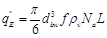(21)

where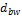is the bubble departure diameter,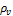, the vapor density,, the active nucleate site density.

These equations need closure for wall boiling parameters:

2.5.1. Bubble Departure Diameter

The default bubble departure diameter (mm) for the RPI model is based on empirical correlations and estimated as: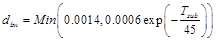(22)

with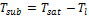is the sub-cooling temperature.

2.5.2. Nucleate Site Density

The nucleate site density is represented by a correlation  based on the wall superheat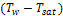as follow: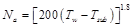(23)

2.5.3. Frequency of Bubble Departure

The bubble departure frequency is calculated as: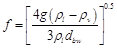(24)

2.5.4. Area Density

The effective wall area occupied by boiling sites definition is based on the departure diameter and the nucleate site density:(25)

where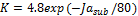, and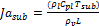is the Jacob number .

2.5.5. Bubble Diameter

The bubble diameter in the free stream is correlated with the local sub-cooling temperature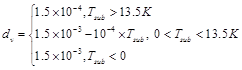(26)

3. CFD Modeling

The commercial CFD code Ansys-Fluent  was used as a frame to perform the simulation. The interfacial forces models and the wall boiling model described previously were implemented in the code through User-Defined Functions (UDFs). The flow domain is shown schematically in Fig. 1. The pipe is 1m in length and 10 mm in diameter. The sub-cooled water enters the system at the bottom, and then boils due to the constant heat flux supplied from the pipe walls.

3.1. Mesh Geometry

Only half of the pipe is considered due to the symmetry at the pipe axis. GAMBIT software was used to build and mesh a two-dimensional computational domain (2-D geometry) with 5x500 uniform rectangular cells.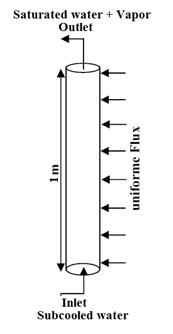Figure 1. Flow domain.

3.2. Initial Boundary Conditions

In the present work, the saturation temperature is fixed to 425.15K. Fully-developed profile of velocity and sub-cooling temperature (Tsub = 5K) is applied at the inlet. The gravity acceleration is 9.8 m/s2. Wall thickness is fixed to 2mm.

3.3. Solution Techniques

Unsteady state calculations with a time step of 0.1 s were fixed for all cases. SIMPLE algorithm was carried out for the calculations of the pressure velocity-coupling and the first order upwind calculation scheme was performed for the discretization of momentum, energy and volume fraction equations.

4. Results and Discussion

The Prediction of axial and radial void fraction distributions and radial temperature profiles for boiling flow in a vertical tube is conducted using the previous described model. Specially seven different wall heat flux are considered from 25 to 150 kWm-2.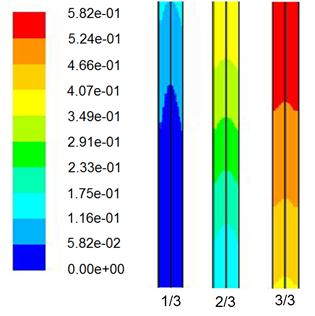Figure 2. Void fraction distribution along the tube.

Fig. 2 illustrates the void fraction distribution along the tube for 90kW/m2. It is seen that no boiling occurs at the inlet zone which corresponds to a single phase heating zone. In fact, the sub-cooled liquid enters the tube, is heated at the wall but vapor is not yet formed at this point. Then, when the sub-cooled water reaches the saturation temperature boiling is initiated.

Fig. 3 depicts the effect of the heat inputs on the void fraction profiles along the pipe axis. One can see that the higher the heat flux is, the lower is the start-up of boiling and the higher is the void fraction. In fact, when the heat input is increased from 25 to 150kW/m2, the onset boiling length is reduced from 43 to 1.6 cm and the void fraction is increased from 0.01 to 0.7 at the tube outlet.

Fig. 4 illustrates radial void fractions at different axial locations for two different heat inputs. One can observe, as expected, that the void fractions are higher adjacent the wall and progressively fall down towards the center for the reason that bubbles form near to the heated wall and then move towards the middle part of the tube.

Radial temperature profiles at different tube locations for 75kW/m2 are depicted in Fig. 5. One can notice that the liquid temperature profiles show the same behavior for the various locations. Actuality, the liquid at the center of the tube is sub-cooled even at the outlet (z = 1 m) and it is superheated near to the wall. We can see also that the sub-cooling level is decreasing throughout the tube’s length.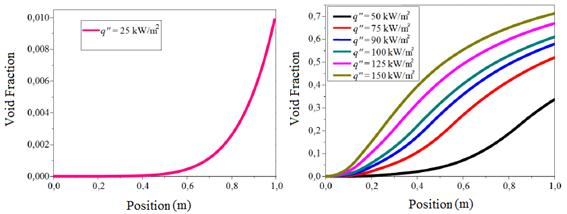Figure 3. Void fraction profiles along the pipe axis for various heat inputs.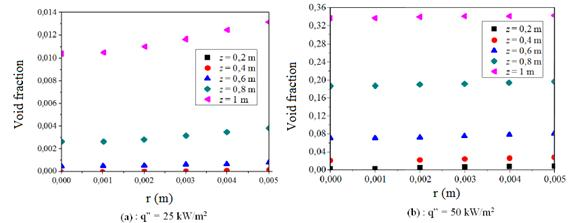Figure 4. Radial void fraction profiles at different axial locations for two different heat inputs.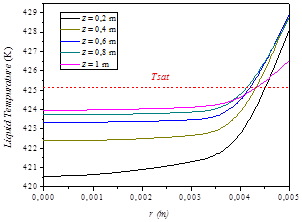Figure 5. Radial liquid temperature profiles at different tube locations.

Liquid and vapor velocities arrangement for 90kW/m2 are illustrated in Fig. 6. One can remark that the liquid velocity increases slightly, it goes from 1.2 to 1.3 m/s from the entrance to 0.4 m, then increases sharply to the rest of the tube it reached 2.9 m/s at the exit. The steam follows almost the same profile as the liquid .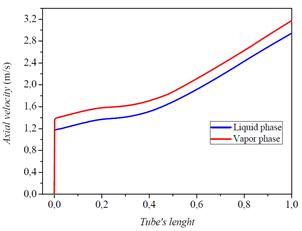Figure 6. Liquid and vapor velocities arrangement.

5. Conclusion

Nucleate boiling flow of water in vertical tube was carried out in this paper using commercial CFD (Computational Fluid Dynamics) package Ansys-Fluent. User defined Functions (UDFs) are employed to model the boiling phenomena. Radial temperature profiles at different tube locations and void fraction distributions for different heat fluxes are calculated and discussed. It was found that the onset boiling point is reduced from 43 to 1.6 cm and the void fraction at tube’s outlet is increased from 0.01 to 0.7 when increasing the wall heat input from 25 to 150kW/m2. The liquid temperature in the middle part of the tube is still sub-cooled along the pipe and it is superheated near to the wall so bubbles migrate from the heated wall to the center of the tube.

References

1. Von Platen BC, Munters CG. US Patent 1, 685,764; 1928.
2. A. Alizadehdakhel, M. Rahimi, A. AbdulazizAlsairaﬁ, CFD modeling of flow and heat transfer in a thermosyphon, International Communications in Heat and Mass Transfer, 37 (2010) 312–318.
3. S. Narumanchi, A. Troshko, D. Bharathan, V. Hassani, Numerical simulations of nucleate boiling in impinging jets: Applications in power electronics cooling, International Journal of Heat and Mass Transfer, 51(2008)1–12.
4. X. Li, R. Wang , R. Huang, Y. Shi, Numerical investigation of boiling flow of nitrogen in a vertical tube using the two-fluid model, Applied Thermal Engineering, 26 (2006) 2425–2432.
5. B. Koncar, I. Kljenak, B. Mavko, Modeling of local two-phase flow parameters in upward subcooled flow boiling at low pressure, International Journal of Heat and Mass Transfer, 47 ( 2004) 1499–1513.
6. N. Kurul, M.Z. Podowski, Multidimensional effects in forced convection subcooled boiling, Ninth International Heat Transfer Conference, Jerusalem, Israel, August 19-24, 1-BO-04 (1990) 21–26.
7. R.M. Podowski, D.A. Drew, R.T.J. Lahey, M.Z. Podowski, A mechanistic model of the ebullition cycle in forced convection subcooled boiling, Eighth International Topical Meeting on Nuclear Reactor Thermal-Hydraulics, Kyoto, Japan, 3 (1997)1535–1542.
8. F.J. Moraga, F.J. Bonetto, R.T. Lahey, Lateral forces on spheres in turbulent uniform shear flow, Int. J. Multiphase Flow, 25 (1999)1321–1372.
9. D.B.R. Kenning, H.T. Victor, Fully-developed nucleate boiling: Overlap of areas of influence and interference between bubble sites, Int. J. Heat Mass Transfer 2 (1981)1025-1032.
10. ANSYS FLUENT Theory Guide. Release 12.0. ANSYS, Inc. April 2009.
11. R. Garma, Y. Stiriba, M. Bourouis, A. Bellagi, Numerical Investigations of the Heating Distribution Effect on the Boiling Flow in the Bubble Pumps, International Journal Of Hydrogen Energy,39(2014) 15256–15260.

 Contents 1. 2. 2.1. 2.2. 2.3. 2.4. 2.5. 3. 3.1. 3.2. 3.3. 4. 5.
Article ToolsAbstractPDF(412K)